JMSLTM Numerical Library 7.2.0
com.imsl.stat

Class CrossCorrelation

• All Implemented Interfaces:
Serializable, Cloneable

public class CrossCorrelation
extends Object
implements Serializable, Cloneable
Computes the sample cross-correlation function of two stationary time series.

CrossCorrelation estimates the cross-correlation function of two jointly stationary time series given a sample of n = x.length observations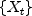and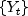for t = 1,2, ..., n.

Let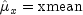be the estimate of the mean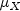of the time serieswhere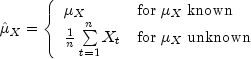The autocovariance function of,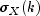, is estimated by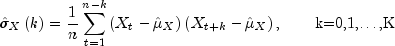where K = maximum_lag. Note that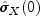is equivalent to the sample variance of x returned by method getVarianceX. The autocorrelation function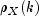is estimated by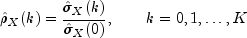Note that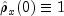by definition. Let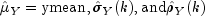be similarly defined.

The cross-covariance function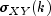is estimated by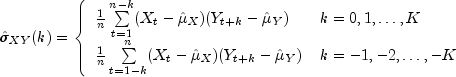The cross-correlation function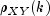is estimated by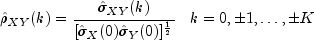The standard errors of the sample cross-correlations may be optionally computed according to the getStandardErrors method argument stderrMethod. One method is based on a general asymptotic expression for the variance of the sample cross-correlation coefficient of two jointly stationary time series with independent, identically distributed normal errors given by Bartlet (1978, page 352). The theoretical formula is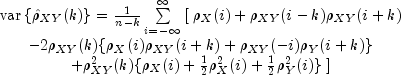For computational purposes, the autocorrelationsand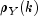and the cross-correlationsare replaced by their corresponding estimates for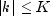, and the limits of summation are equal to zero for all k such that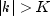.

A second method evaluates Bartlett's formula under the additional assumption that the two series have no cross-correlation. The theoretical formula is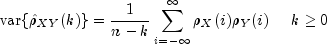For additional special cases of Bartlett's formula, see Box and Jenkins (1976, page 377).

An important property of the cross-covariance coefficient isfor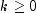. This result is used in the computation of the standard error of the sample cross-correlation for lag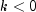. In general, the cross-covariance function is not symmetric about zero so both positive and negative lags are of interest.

Example, Serialized Form
• Field Summary

Fields
Modifier and Type Field and Description
static int BARTLETTS_FORMULA
Indicates standard error computation using Bartlett's formula.
static int BARTLETTS_FORMULA_NOCC
Indicates standard error computation using Bartlett's formula with the assumption of no cross-correlation.
• Constructor Summary

Constructors
Constructor and Description
CrossCorrelation(double[] x, double[] y, int maximum_lag)
Constructor to compute the sample cross-correlation function of two stationary time series.
• Field Detail

• BARTLETTS_FORMULA

public static final int BARTLETTS_FORMULA
Indicates standard error computation using Bartlett's formula.
Constant Field Values
• BARTLETTS_FORMULA_NOCC

public static final int BARTLETTS_FORMULA_NOCC
Indicates standard error computation using Bartlett's formula with the assumption of no cross-correlation.
Constant Field Values
• Constructor Detail

• CrossCorrelation

public CrossCorrelation(double[] x,
double[] y,
int maximum_lag)
Constructor to compute the sample cross-correlation function of two stationary time series.
Parameters:
x - A one-dimensional double array containing the first stationary time series.
y - A one-dimensional double array containing the second stationary time series.
maximum_lag - An int containing the maximum lag of the cross-covariance and cross-correlations to be computed. maximum_lag must be greater than or equal to 1 and less than the minimum of the number of observations of x and y.
• Method Detail

• getAutoCorrelationX

public double[] getAutoCorrelationX()
throws CrossCorrelation.NonPosVariancesException
Returns the autocorrelations of the time series x.
Returns:
A double array of length maximum_lag +1 containing the autocorrelations of the time series x. The 0-th element of this array is 1. The k-th element of this array contains the autocorrelation of lag k where k = 1, ..., maximum_lag.
Throws:
CrossCorrelation.NonPosVariancesException
• getAutoCorrelationY

public double[] getAutoCorrelationY()
throws CrossCorrelation.NonPosVariancesException
Returns the autocorrelations of the time series y.
Returns:
A double array of length maximum_lag +1 containing the autocorrelations of the time series y. The 0-th element of this array is 1. The k-th element of this array contains the autocorrelation of lag k where k = 1, ..., maximum_lag.
Throws:
CrossCorrelation.NonPosVariancesException
• getAutoCovarianceX

public double[] getAutoCovarianceX()
throws CrossCorrelation.NonPosVariancesException
Returns the autocovariances of the time series x.
Returns:
A double array of length maximum_lag +1 containing the variances and autocovariances of the time series x. The 0-th element of the array contains the variance of the time series x. The k-th element contains the autocovariance of lag k where k = 1, ..., maximum_lag.
Throws:
CrossCorrelation.NonPosVariancesException
• getAutoCovarianceY

public double[] getAutoCovarianceY()
throws CrossCorrelation.NonPosVariancesException
Returns the autocovariances of the time series y.
Returns:
A double array of length maximum_lag +1 containing the variances and autocovariances of the time series y. The 0-th element of the array contains the variance of the time series x. The k-th element contains the autocovariance of lag k where k = 1, ..., maximum_lag.
Throws:
CrossCorrelation.NonPosVariancesException
• getCrossCorrelation

public double[] getCrossCorrelation()
throws CrossCorrelation.NonPosVariancesException
Returns the cross-correlations between the time series x and y.
Returns:
A double array of length 2 * maximum_lag +1 containing the cross-correlations between the time series x and y. The cross-correlation between x and y at lag k, where k = -maximum_lag ,..., 0, 1,...,maximum_lag, corresponds to output array indices 0, 1,..., (2*maximum_lag).
Throws:
CrossCorrelation.NonPosVariancesException
• getCrossCovariance

public double[] getCrossCovariance()
Returns the cross-covariances between the time series x and y.
Returns:
A double array of length 2 * maximum_lag +1 containing the cross-covariances between the time series x and y. The cross-covariance between x and y at lag k, where k = -maximum_lag ,..., 0, 1,...,maximum_lag, corresponds to output array indices 0, 1,..., (2*maximum_lag).
• getMeanX

public double getMeanX()
Returns the mean of the time series x.
Returns:
A double containing the mean of the time series x.
• getMeanY

public double getMeanY()
Returns the mean of the time series y.
Returns:
A double containing the mean of the time series y.
• getStandardErrors

public double[] getStandardErrors(int stderrMethod)
throws CrossCorrelation.NonPosVariancesException
Returns the standard errors of the cross-correlations between the time series x and y. Method of computation for standard errors of the cross-correlation is determined by the stderrMethod parameter. If stderrMethod is set to BARTLETTS_FORMULA, Bartlett's formula is used to compute the standard errors of cross-correlations. If stderrMethod is set to BARTLETTS_FORMULA_NOCC, Bartlett's formula is used to compute the standard errors of cross-correlations, with the assumption of no cross-correlation.
Parameters:
stderrMethod - An int specifying the method to compute the standard errors of cross-correlations between the time series x and y.
Returns:
A double array of length 2 * maximum_lag + 1 containing the standard errors of the cross-correlations between the time series x and y. The standard error of cross-correlations between x and y at lag k, where k = -maximum_lag,..., 0, 1,..., maximum_lag, corresponds to output array indices 0, 1,..., (2*maximum_lag).
Throws:
CrossCorrelation.NonPosVariancesException
• setMeanX

public void setMeanX(double mean)
Estimate of the mean of time series x.
Parameters:
mean - A double containing the estimate mean of the time series x.
• setMeanY

public void setMeanY(double mean)
Estimate of the mean of time series y.
Parameters:
mean - A double containing the estimate mean of the time series y.
JMSLTM Numerical Library 7.2.0